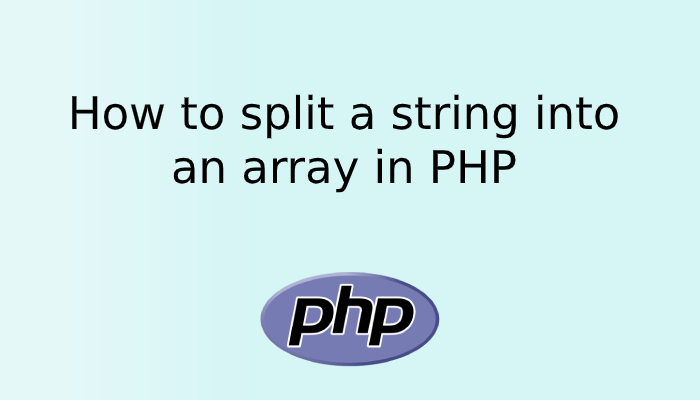# How to split a string into an array in PHPSometimes we need to divide the string into an array. For example, we have a paragraph or we are getting values from the database in string format which consists of multiple words with comma-separated or hyphen separated. In that situation sometimes we need to divide the string into an array. So in this post, we will understand how to split a string into an array in PHP.

PHP provides us a explode() functions that can split a string into an array. The explode() function splits a string based on a string delimiter, for example, if we need to split the string on the basis of comma so we need to pass the comma in the function. This function returns an array containing the string separated by delimiters.

explode() function split the or break a string into an array by a separator like a comma, space, hyphen, etc. You can also set the optional parameter to specify the number of return elements in an array.

Syntax :

``````array explode(separator, mainString, numberOfElements)
``````

Parameters:  We can pass three parameters in explode() function in which the first two are compulsory and the third one is optional, you can pass the third parameter as per requirement.

We have tried to explain are three parameters below.

1. separator: This parameter act as a separator as we define and tell the function to divide the string from this parameter found in the string. i.e. whenever the function found this character, it split the string from there and convert into an array.
2. mainString: The main string.
3. numberOfElements: This is an optional parameter, you have to choose to pass it or let it keep blank. It is used to specify the number of elements of the array. This parameter can be any integer ( positive, negative, or zero)

Let’s check the below example and see how it works.

```<?php
\$my_str = 'Hello welcome in my blog and you are learning php';
\$new_array= explode(" ", \$my_str);
print_r(\$new_array);

print_r(explode(" ", \$my_str, 4));

?>```

Output

``````Array
(
 => Hello
 => welcome
 => in
 => my
 => blog
 => and
 => you
 => are
 => learning
 => php
)
Array
(
 => Hello
 => welcome
 => in
 => my blog and you are learning php
)``````

In the above example, we have defined \$my_str which consists of strings with space. While using explode() function we have passed space as a first parameter and \$my_str variable as a second parameter. So now explode() function will break the \$my_str variable into an array based on the first parameter i.e. based on space.

So wherever explode() function will get space in the \$my_str variable it will break that string into an array. You can check the final output.

In the First example strings get divided into an array based on space and in second example it also gets divided bases on the first parameter but returns only 4 value because we have passed 4 in the third parameter in explode() function. So the third parameter is optional and you only need to use that whenever you need to get a specific number of array values.

## Conclusion:

In the above post, you got to know how easy is to split the string into an array using PHP using explode() function. explode function is widely used in the PHP projects as it is very easy to use and most of the time we know we need to convert a string into an array.

Hope you liked the post, you can also check How to Convert a String to Array Using PHP Without Explode Function?.